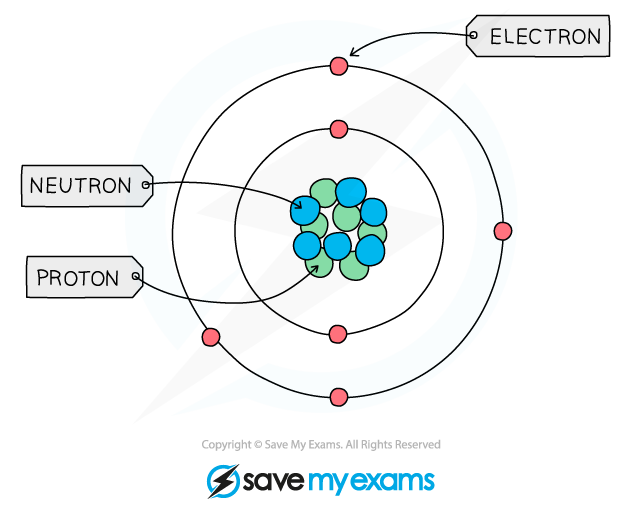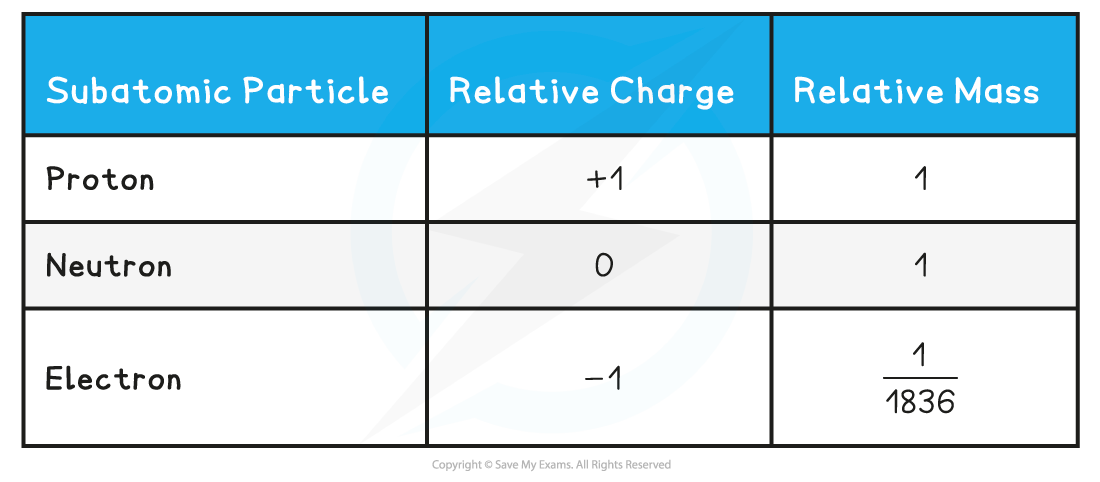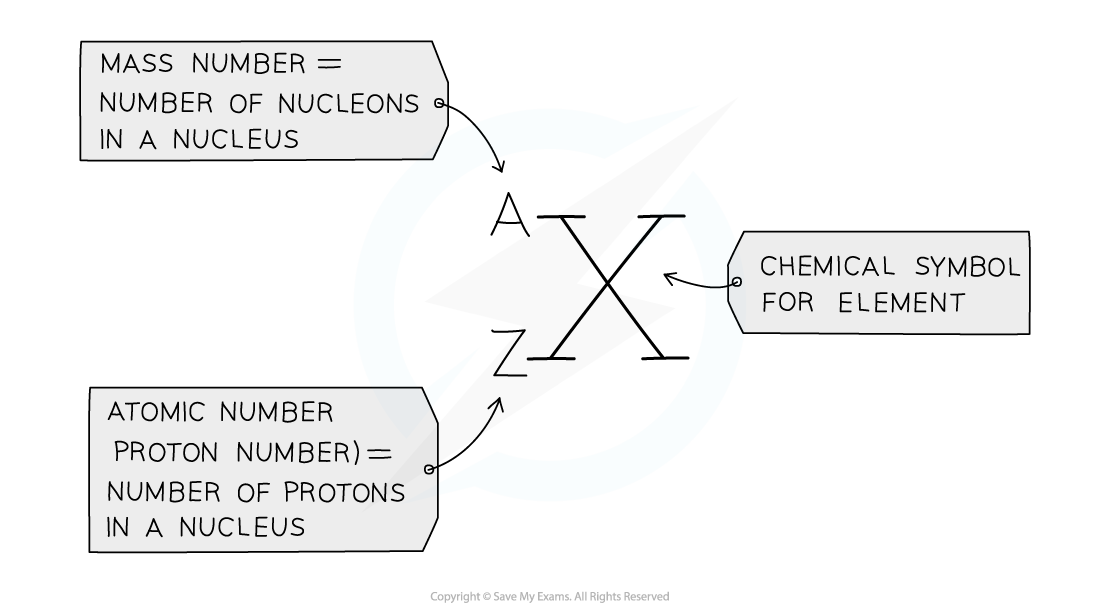# AQA A Level Chemistry复习笔记1.1.1 Fundamental Particles

### Structure of an Atom

• All matter is composed of atoms, which are the smallest parts of an element that can take place in chemical reactions
• Atoms are mostly made up of empty space around a very small, dense nucleus that contains protons and neutrons
• The nucleus has an overall positive charge
• The protons have a positive charge and the neutrons have a neutral charge

• Negatively charged electrons are found in orbitals in the empty space around the nucleusThe basic structure of an atom (not to scale)

### Subatomic Particles

• Subatomic particles are the particles an element is made up of and include protons, neutrons and electrons
• These subatomic particles are so small that it is not possible to measure their masses and charges using conventional units (such as grams and coulombs)
• Instead, their masses and charges are compared to each other using ‘relative atomic masses’ and ‘relative atomic charges’
• These are not actual charges and masses but they are charges and masses of particles relative to each other
• Protons and neutrons have a very similar mass so each is assigned a relative mass of 1 whereas electrons are 1836 times smaller than a proton and neutron
• Protons are positively charged, electrons negatively charged and neutrons are neutral

• The relative mass and charge of the subatomic particles are:

Relative mass & charge of subatomic particles table#### Exam Tip

The relative mass of an electron is almost negligible.The charge of a single electron is -1.602 x 10-19  coulombs whereas the charge of a proton is +1.602 x 10-19  coulombs, however, relative to each other, their charges are -1 and +1 respectively.

### Atoms: Key Terms

• The atomic number (or proton number) is the number of protons in the nucleus of an atom and has symbol Z
• The atomic number is equal to the number of electrons present in a neutral atom of an element
• Eg. the atomic number of lithium is 3 which indicates that the neutral lithium atom has 3 protons and 3 electrons

• The mass number (or nucleon number) is the total number of protons and neutrons in the nucleus of an atom and has symbol A
• The number of neutrons can be calculated by:

Number of neutrons = mass number - atomic number

• Protons and neutrons are also called nucleons

#### Exam TipThe mass (nucleon) and atomic (proton) number are given for each element in the Periodic Table